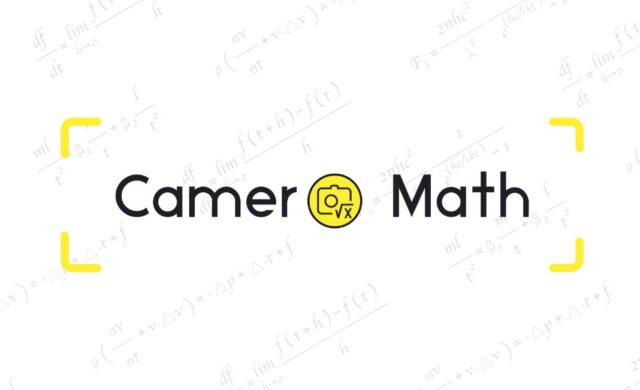Home Blog The Convenient Math Scientific Calculator You Can’t Miss – Math Solver in...

# The Convenient Math Scientific Calculator You Can’t Miss – Math Solver in Cameramath## What is CameraMath’s Math Solver?

CameraMath is a free Math Solver for cell phones with picture solving, tutor lookup, calculator, and other features. Math Solver is a built-in problem-solving tool on CameraMath’s official website. It mainly focuses on the calculation of mathematical equations and helps users to get the answers quickly with detailed instructions on how to make them. It can solve many types of equations and the team is still improving this solver, CameraMath team built it to facilitate the use of scientific computing for computer users.

## What types of problems can you use to solve?

Math Solvers can solve many types of problems, specifically:

– Algebra: Equations, Expressions, Inequalities, Functions, Sequences, Log arithmetic, Complex Numbers, Matrices

– Calculus: Derivatives, Integrals, Limits, Series

Trigonometry: Equations, Inequalities, Functions, Simplify

– Matrix

## How to use the Math Solver in CameraMath?

First, go to the CameraMath website, find Math Solver in the upper left corner of the navigation, and click on Math Solver to see the calculator.

Determine the type of math problem you want to solve, and then select the appropriate calculator from the navigation above the math keyboard, as shown in the figure. There are four main types of calculators: algebra, calculus, trigonometry, and matrices.

Next, you just need to enter the equation you want to solve. Note that the different types of calculators are not independent of each other and can be switched between them, and the keyboard provided by the website can be used together with the computer keyboard.

After entering the equation, click on the solve button to get the corresponding result and explain the steps. If the result of the answer can be displayed by images or other forms, the Math Solver will also display it together.

The above description and diagrams allow us to summarize the advantages and disadvantages of Math Solver.

### Pros:

– Free

– Easy to use

– Provides detailed solutions

– Hardware keyboard support

– Examples to help get started quickly

### Cons:

– Not a lot of “complicated features”

– The detailed process requires a membership version to view